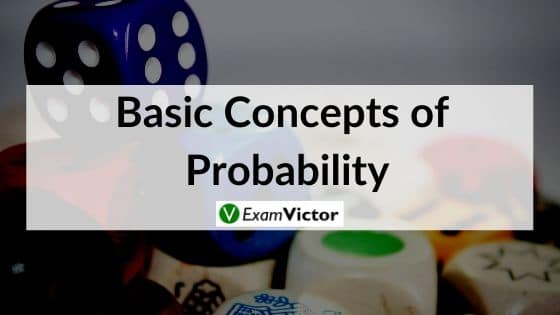# Basic Concepts of ProbabilityLast Updated on Nov 5, 2020

Many events can’t be predicted with total certainty. The best we can say is how likely they are to happen, using the idea of probability.

For example,

1) When a coin is tossed, there are two possible outcomes each with the probability of 1/2.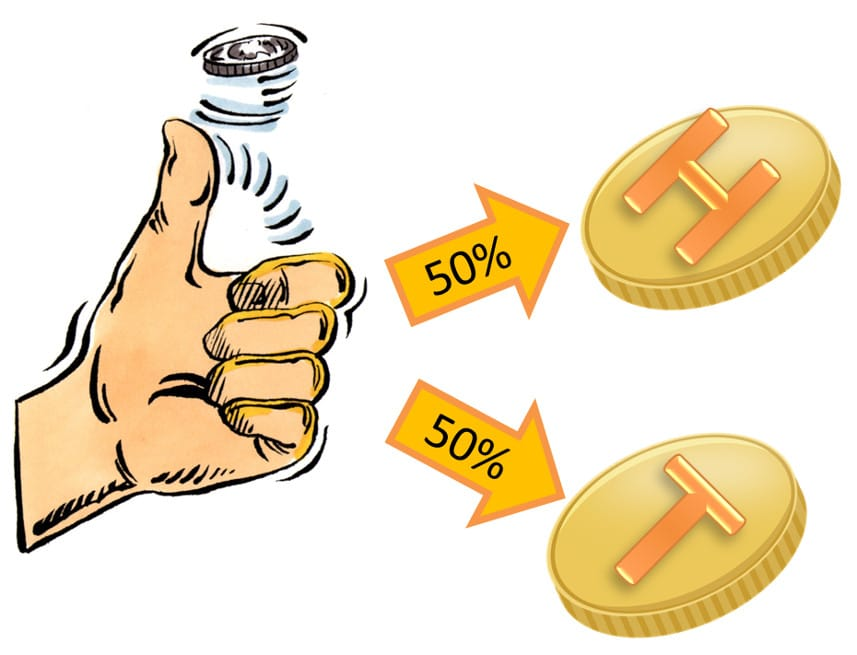2) When a single die is thrown, there are six possible outcomes: 1, 2, 3, 4, 5, 6.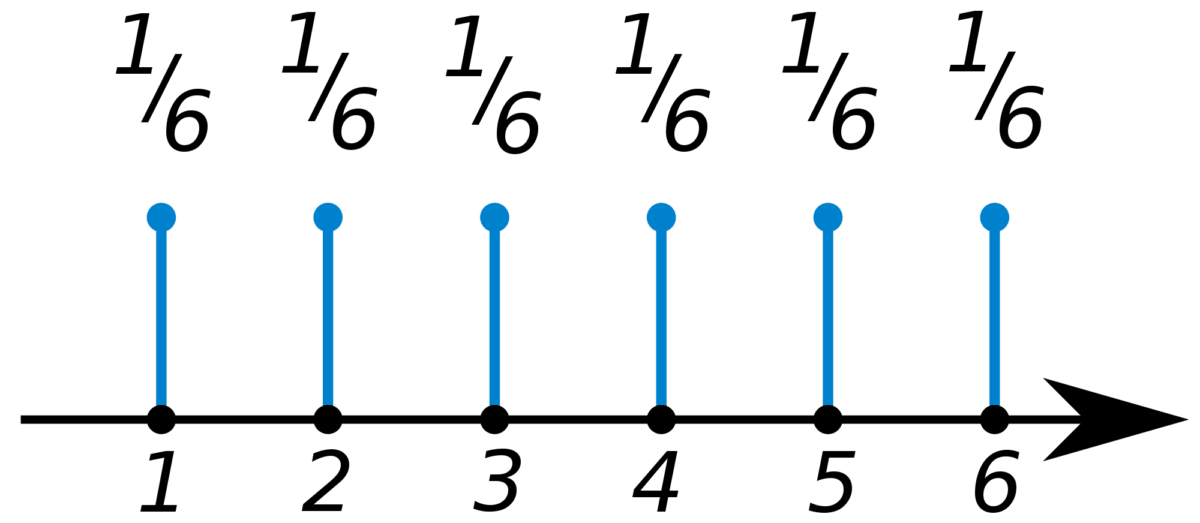In general, Probability is:Example: A spinner has 4 equal sectors colored yellow, blue, green and red. After spinning the spinner, what is the probability of landing on each color?

The possible outcomes of this experiment are yellow, blue, green, and red.

Probabilities: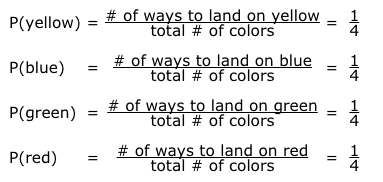##### Probability of Mutually Exclusive Events

Two events are said to be mutually exclusive if they cannot happen at the same time.
For example, if we toss a coin, either heads or tails might turn up, but not heads and tails at the same time. Similarly, in a single throw of a die, we can only have one number shown at the top face. The numbers on the face are mutually exclusive events.

If A and B are mutually exclusive events then the probability of A happening OR the probability of B happening is P(A) + P(B).

P(A or B) = P(A) + P(B)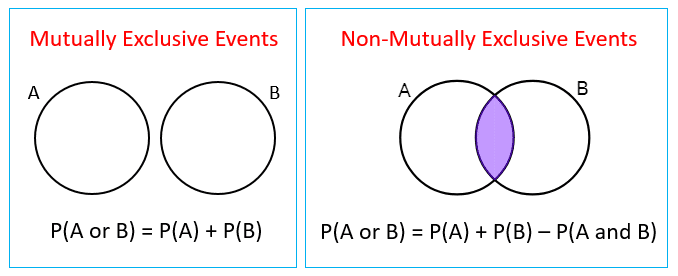Example: What is the probability of a die showing a 2 or a 5?

Solution: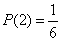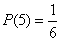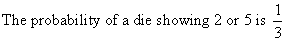What are Independent Events in Probability?
Events are independent if the outcome of one event does not affect the outcome of another. For example, if you throw a die and a coin, the number on the die does not affect whether the result you get on the coin.

How to calculate the probability of independent events?

If A and B are independent events, then the probability of A happening AND the probability of B happening is P(A) × P(B).

The following gives the multiplication rule to find the probability of independent events occurring together. Scroll down the page for more examples and solutions of word problems that involve the probability of independent events.

Example: If a dice is thrown twice, find the probability of getting two 5’s.

Solution: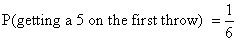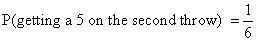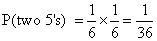### Summary

In this post, we have explained the concept of Probability in depth with illustrations which are easy to understand to clarify the concepts.

Let us know your suggestions in the comment section below.